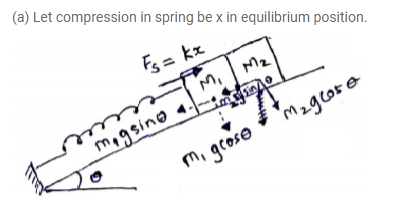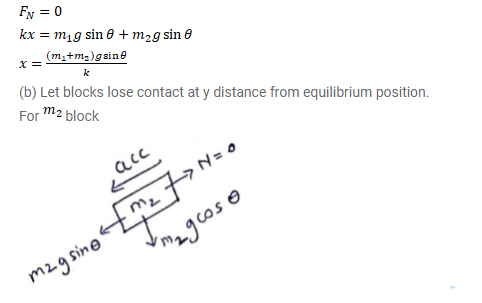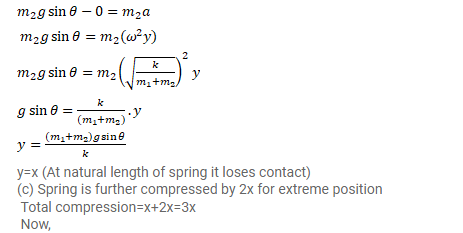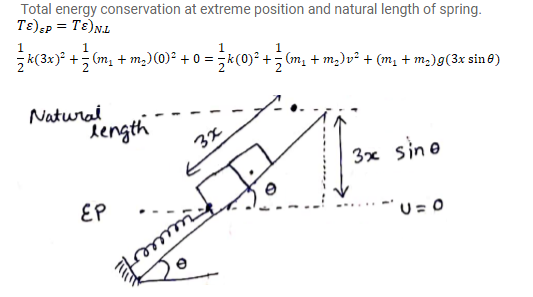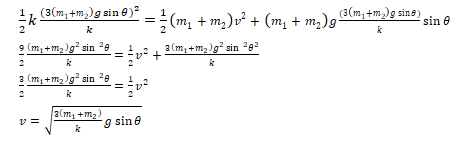# The block of mass m1 shown in figure is fastened to the spring and the block of

Question:

The block of mass $m_{1}$ shown in figure is fastened to the spring and the block of mass $m_{2}$ is placed against it.

(a) Find the compression of the spring in the equilibrium position.

(b) The blocks are pushed a further distance $\left(\frac{2}{k}\right)\left(m_{1}+m_{2}\right) g \sin \theta$ against the spring and released. Find the position where the two blocks separate.

(c) What is common speed of blocks at the time of separation?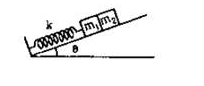Solution: